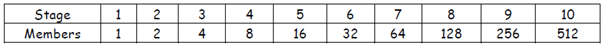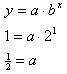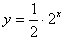Exponential Functions

Exponential functions are written in the form: y = abx, where b is the constant ratio and a is the initial value. By examining a table of ordered pairs, notice that as x increases by a constant value, the value of y increases by a common ratio. This is characteristic of all exponential functions.

In the table below notice that as the x-values increase by 1, the y-values double. In other words, to get from one y-value to the next, multiply by 2, therefore the common ratio is 2.To find the equation that represents this table of values, substitute any ordered pair from the table into the equation, and solve for a.So the model for this data is:Listen